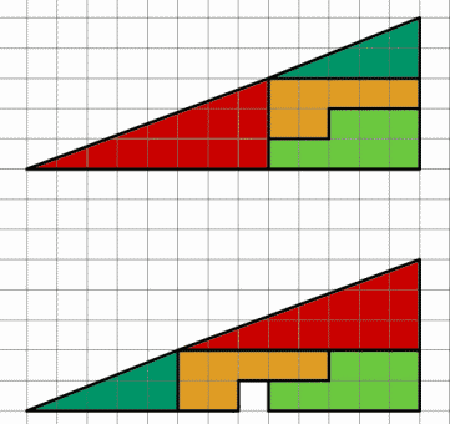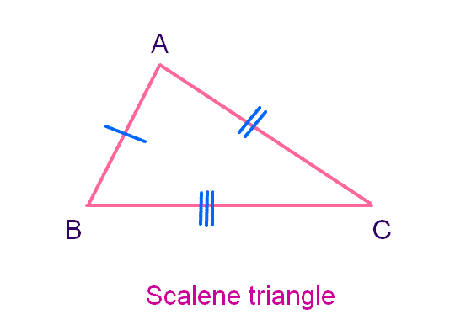## Types of Triangles

Published on: Mar 01 2011 by Shenron

A triangle is one of the basic geometry figures which is a polygon having three sided shape that lies in one plane. We can define triangle as a polygon with three sides and three angles. Note that the sum of all the angles would be 180º. Each triangle has three corners or vertices, a base (any of the three sides) and an altitude (perpendicular height from base to the opposite vertex).

In Euclidean geometry, triangle is one of the basic figures. Three fundamental elements are essential to make a triangle, i.e.• 3-sided
• Plane or two-dimensional figure
• The sum of the all the angles should be exactly 180 degrees.

Triangles are classified into different types on the basis of two classification schemes. The first classification scheme indicates three types of triangle on the basis of its sides.

Equilateral Triangle: Equilateral literally means ‘equal sides’. Therefore, in an equilateral triangle all three sides have same length and the angles are also equal, i.e. 60°. This feature makes the triangular equiangular.

Isosceles Triangle: Isosceles triangles are the triangles which possess two equal sides. The angles formed at equal sides must be equal at the point where these equal sides meet the third side.

Scalene Triangle: Scalene triangles are the triangles which have unequal sides and angles. In these types of triangle, all the sides are of different length and hence, the angles are also not equal.

The second classification scheme is the one which focuses on the measurement of the internal angles.Acute Triangle: Acute triangle is the one which its largest internal angle less than 90° or acute. So, we can conclude that in acute triangle, the measurement of all the three angles are less than 90°.

Other Triangles: The term triangle is not limited to Euclidean geometry as the term is often used to refer to things that are similar to triangle in their appearances, i.e. they either have shapes similar to triangle or possess three sides connected with in a similar fashion as that of triangle. For instance, a musical instrument is named as triangle because of its metal like shape similar to triangle. However, the one end of the instrument is open. Triangular beater is used to hit the thin wire. The instrument is used for band music and orchestral.

The term triangle is also used to refer to the places having shape similar to triangle. For instance,

The Bermuda Triangle often indicated by Bermuda, Fort Lauderdale and Puerto Rico. Similarly, there are several different Golden Triangles comprising of the regions of Southeast Asia known for opium production. Golden Triangle is often considered as isosceles triangle appears so that the ratio of the sides or hypotenuse to the base is equal to the said golden ratio.